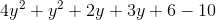Example Questions

Example Question #1 : How To Add Trinomials

Add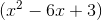and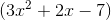.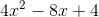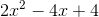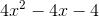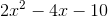Explanation:

To add the trinomials, simply eliminate the parentheses and add like terms.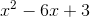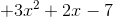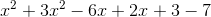Example Question #2 : How To Add Trinomials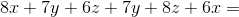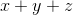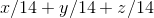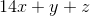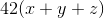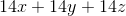Explanation:

Like terms can be added together: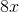is added to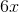,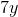is added to, and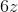is added to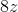. The resulting answer choice that is correct is.

Example Question #3 : How To Add Trinomials

Choose the answer which best simplifies the following expression: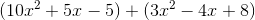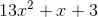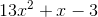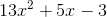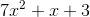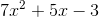Explanation:

To solve this problem simply remove the parentheses and add the like terms: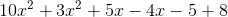Example Question #4 : How To Add Trinomials

Choose the answer which best simplifies the following expression: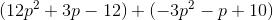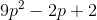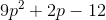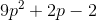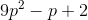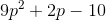Explanation:

To simplify, remove parentheses and combine like terms: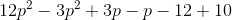Example Question #5 : How To Add Trinomials

Choose the answer which best simplifies the following expression: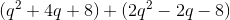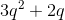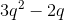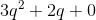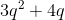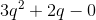Explanation:

To simplify, remove parentheses and combine like terms: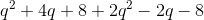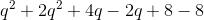Note that adding or subtracting a zero to the end of this equation is unnecessary.

Example Question #6 : How To Add Trinomials

Choose the answer which best simplifies the following expression: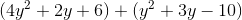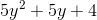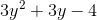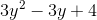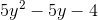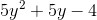Explanation:

To simplify, simply remove the parentheses and combine like terms: